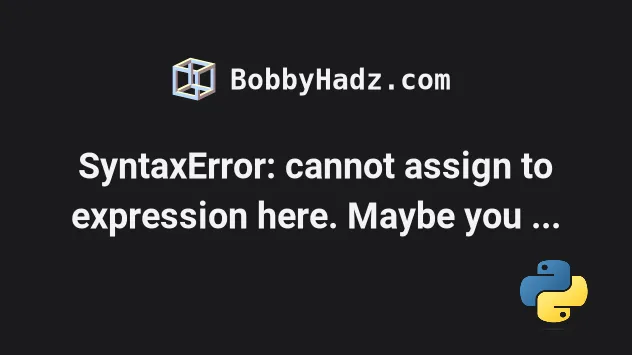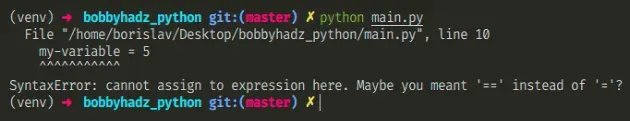# SyntaxError: cannot assign to expression here. Maybe you meant '==' instead of '='?Mon Apr 25 20222 min read## SyntaxError: cannot assign to expression here. Maybe you meant '==' instead of '='?#

The Python "SyntaxError: cannot assign to expression here. Maybe you meant '==' instead of '='?" occurs when we have an expression on the left-hand side of an assignment. To solve the error, specify the variable name on the left and the expression on the right-hand side.Here is an example of how the error occurs.

main.py
```Copied!```# ⛔️ SyntaxError: cannot assign to expression here. Maybe you meant '==' instead of '='?
my-variable = 5
``````
We have a hyphen `-` in the name of the variable, so Python thinks we are trying to subtract two variables.

If this is how you got the error, use an underscore instead of a hyphen.

main.py
```Copied!```my_variable = 5

print(my_variable)  # 👉️ 5
``````

The name of a variable must start with a letter or an underscore.

A variable name can contain alpha-numeric characters (`a-z`, `A-Z`, `0-9`) and underscores `_`.

Variable names cannot contain any other characters than the aforementioned.

Here is another example of how the error occurs.

main.py
```Copied!```a = 20
b = 5

# ⛔️ SyntaxError: cannot assign to expression here. Maybe you meant '==' instead of '='?
a/b = 4
``````

We have an expression on the left-hand side which is not allowed.

The variable name has to be specified on the left-hand side, and the expression on the right-hand side.
main.py
```Copied!```a = 20
b = 5

result = a / b
print(result)  # 👉️ 4.0
``````

If you mean to compare two values, use double equals sign.

main.py
```Copied!```a = 20
b = 5

if a/b == 4:
# 👇️ this runs
print('Success')
else:
print('Failure')
``````

Notice that we use double equals `==` when comparing two values and a single equal `=` sign for assignment.

If you got the error when declaring a variable that stores a dictionary, use the following syntax.

main.py
```Copied!```my_dict = {'name': 'Alice', 'age': 30, 'tasks': ['dev', 'test']}
``````

Notice that each key and value are separated by a colon and each key-value pair is separated by a comma.

Use the search field on my Home Page to filter through my more than 1,000 articles.